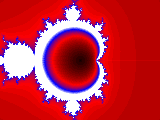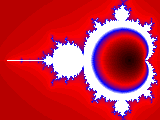PHOTOSWIZZLE Fractals Help - Position## Position

The position controls are for up-down (V, vertical) and right-left (H, horizontal) movement. The position determines the image center point, and all the other controls operate with respect to the center. The position range is from -9999 to 9999 so the position may be negative as well as positive. With such a large position range, moving the slider can become a problem sometimes. See range for details about how the range control can help make zoom easier to work with.

The position controls seem pretty straightforward to understand at first. But sometimes when using them you might find that they appear to be going the "wrong way". That happens because of a characteristic of the X-Y Rotation control. Fractals has to do some calculations for all of the control settings, and at some point a decision had to be made on what order to do them in. The order is fixed and the position is applied before X-Y Rotation.

For example suppose the X-Y Rotation is set to 90. Then you move the H Position but the image appears to move vertically instead. This is because Fractals calculates the H Position movement first, and then the rotation so it looks like the image is moving vertically. So if things appear to be going to wrong way, try to remember to look at the X-Y Rotation and you'll find that it's set to something other than 0 and at least then you'll know what's going on. The recommended way to keep things straight is to set X-Y Rotation to 0, set all of the other controls where you want them, then do the X-Y Rotation last.

Here's an example of two different H position settings. The image on the right was created with the Fractals default settings. The image on the left has the same settings except for H position 0. This shows how the fractal equation doesn't necessarily always come up with a visually pleasing image by default and that's where your creativity and subjective view are really important.H Position = 0H Position = 60

```
```